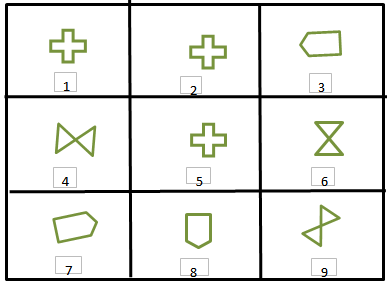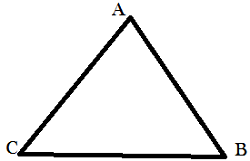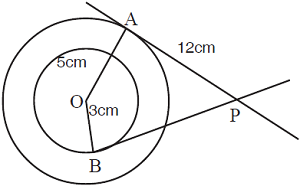# CREST Olympiads - CMO PDF Sample Papers for Class 9

Resources:

Class 9 sample paper & practice questions for CREST Mathematics Olympiad (CMO) level 1 are given below. Syllabus for level 1 is also mentioned for these exams. You can refer these sample paper & quiz for preparing for the CMO exam.Q.1 Q.2 Q.3 Q.4 Q.5 Q.6 Q.7 Q.8 Q.9 Q.10
 Q.1 The difference between the first two perfect squares that end with a 9 is: a) 11 b) 40 c) 30 d) 120
 Q.2 Find the equation of the straight line joining the points of intersection of 2x + y = 4 with x - y + 1 = 0 and 2x - y - 1 = 0 with x + y - 8 = 0. a) x + 2y = 3 b) x + 2y + 3 = 0 c) x - 2y + 3 = 0 d) 3x - 2y + 1 = 0
 Q.3 It takes Riaz 30 minutes to mark a paper. Razi only need 25 minutes to mark a paper. If they both start marking paper at 11:00 AM, What is the first time they will finish marking a paper at the same time? a) 12:30 b) 12:45 c) 1:30 d) 12:25
 Q.4 What is the next number in this sequence: Identify the identical figures from the following options given.a) 1,2,5; 3,8,9; 4,6,7 b) 1,2,5; 4,7,8; 5,6,9 c) 1,2,5; 3,7,8; 4,6,9 d) 1,3,5; 2,7,8; 4,6,9
 Q.5 If a circular metal sheet is 0.65 cm thick and of 50 cm in diameter is melted and recast into cylindrical bar with 8 cm diameter then length of bar will be: a) 25.41 cm b) 35.41 cm c) 40.41 cm d) 25.39 cm
 Q.6 In ΔABC if AB = BC, then:a) ∠B > ∠C b) ∠A = ∠C c) ∠A = ∠B d) ∠A < ∠B
 Q.7 If surface area of sphere is 144π cm². What is its radius? a) 5 cm b) 6 cm c) 7 cm d) 8 cm
 Q.8 What is the length of the largest rod that can be put in a box of inner dimensions 30 cm, 24 cm and 18 cm? a) 20 √2 cm b) 50 cm c) 60 cm d) 30 √2 cm
 Q.9 Find BP.a) 4 √10 cm b) 5√10 cm c) 6√10 cm d) 7√10 cm
 Q.10 The volume of a cube is 1000 cm3. What will be the length of its edge? a) 10 cm b) 100 cm c) 1000 cm d) 0.1 cmSample PDF of CREST Olympiads - CREST Mathematics Olympiad (CMO) PDF Sample Papers for Class 9: